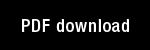A prediction theory for some nonlinear functional-differential equations, I: Learning of lists

Author(s): Grossberg, S. |

Year: 1968

Citation: Journal of Mathematical Analysis and Applications, 21, 643-694

Abstract: In this paper, we study some systems of nonlinear functional-differentialequations of the form
X(t) = AX(t) + B(X,) X(t - r) + C(t), t > 0, (1)which were introduced in Grossberg (, (2], ).
We will choose (l) sothat X = (xl , x2 ,..., x,,) is nonnegative, B(X,) = 11 Bjj(t) 11 is a matrix ofnonnegative and nonlinear functionals of X(w) evaluated at all past timesw E [- r, t], and C = (1, , 4 ,..., Iâ€ž) is a known nonnegative and continuousinput function. We will show that for appropriate choices of A, B, and C,ratios such asXk(t) = xk(() xm(t))-1M-L
have limits as t --? co, for all j, k = 1, 2,..., n.For these choices of A, B, and C, we will be able to interpret (1) as aprediction theory. The goal of this theory is to discuss the prediction ofindividual events, in a fixed order, and at prescribed times. The theory is nothomogeneous in time. A system which produces random predictions at t = 0can be gradually transformed into a system whose predictions become deter-ministic as t --- oo. Similarly, a system which produces deterministic pre-dictions at t == 0 can be gradually transformed into a system whose predic-tions become random as t -. co. The factor which primarily determines if asystem becomes random or deterministic in its predictions as t -? co is thesystem's input function C(t). C(t) is the "environment" or "experience" ofthe system, and we will make precise the statement that these systems "adaptto their environment" or "learn from experience."
Our systems can also be interpreted as cross-correlated flows on networks,or as deformations of probabilistic graphs. They often have the propertythat the average input [...] is related to the average output [...] through a system of linear difference-differential equations. This propertyis crucial to our proofs. Another important property is the nonnegativity ofinitial data. When mixtures of positive and negative initial data are chosen,the results are not true in general.

Topics: Mathematical Foundations of Neural Networks,